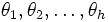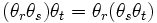# Historical definitions of group

Refer history of groups for more about the history of the definition and concept of group, and funky definitions of group for a list of weird definitions of group.

## Definitions using permutations

### Jordan

In a paper in 1869 titled Commentaire sur Galois, Jordan defines a group as follows:

A system of substitutions forms a group if the product of any two substitutions of the system is again a member of the system.

In his book Traité des substitutions, Jordan defines a permutation (he calls it a substitution) and then goes on to define a group:

We will say that a system of substitutions form a group if the product of any two substitutions in the system is itself in the system.

## Definitions in the abstract setting

### Cayley (1854)

Cayley attempted the first general definition of a group, in his paper On the theory of groups, as depending on the symbolic equation$\theta^n = 1$. This definition is not precisely the same as the modern definition, though it is equivalent. Cayley states:

A set of symbols$1, \alpha, \beta, \dots$, all of them different, and such that the product of any two of them (no matter in what order), or the product of any one of them into itself, belongs to the set, is said to be a group. These symbols are not in general convertible (commutative) but associative, it follows that if the entire group is multiplied by any one of the symbols, either as further or nearer factor (left or right), the effect is simply to reproduce the group.

This definition is today summarized as saying that a group is an associative quasigroup. It is equivalent to the modern definition of group. Further information: Associative quasigroup equals group

### Weber (1882)

Heinrich Weber gave this definition in a paper on quadratic forms.

A system$G$ of$h$ arbitrary elements$\theta_1, \theta_2, \dots, \theta_h$ is called a group of degree$h$ if it satisfies the following conditions:

I. By some rule which is designated as composition or multiplication, from any two elements of the same system one derives a new element of the same system.
II. It is always true that$(\theta_r \theta_s)\theta_t = \theta_r(\theta_s\theta_t)$.

III. From$\theta \theta_r = \theta \theta_s$ it follows that$\theta_r = \theta_s$.

Weber's definition is equivalent to the modern definition of finite group.